PyTorch自定义CUDA算子教程与运行时间分析

https://github.com/godweiyang/torch-cuda-example

## 完整流程

• main.py，这是python入口，也就是你平时写模型的地方。
• add2.cpp，这是torch和CUDA连接的地方，将CUDA程序封装成了python可以调用的库。
• add2.h，CUDA函数声明。
• add2.cu，CUDA函数实现。

### CUDA算子实现

void launch_add2(float *c,
const float *a,
const float *b,
int n);
__global__ void add2_kernel(float* c,
const float* a,
const float* b,
int n) {
for (int i = blockIdx.x * blockDim.x + threadIdx.x; \
i < n; i += gridDim.x * blockDim.x) {
c[i] = a[i] + b[i];
}
}

const float* a,
const float* b,
int n) {
dim3 grid((n + 1023) / 1024);
dim3 block(1024);
}

add2_kernel是kernel函数，运行在GPU端的。而launch_add2是CPU端的执行函数，调用kernel。注意它是异步的，调用完之后控制权立刻返回给CPU，所以之后计算时间的时候要格外小心，很容易只统计到调用的时间。

### Torch C++封装

#include <torch/extension.h>

const torch::Tensor &a,
const torch::Tensor &b,
int n) {
(const float *)a.data_ptr(),
(const float *)b.data_ptr(),
n);
}

PYBIND11_MODULE(TORCH_EXTENSION_NAME, m) {
}

torch_launch_add2函数传入的是C++版本的torch tensor，然后转换成C++指针数组，调用CUDA函数launch_add2来执行核函数。

### Python调用

import time
import numpy as np
import torch

verbose=True)

# c = a + b (shape: [n])
n = 1024 * 1024
a = torch.rand(n, device="cuda:0")
b = torch.rand(n, device="cuda:0")
cuda_c = torch.rand(n, device="cuda:0")

ntest = 10

def show_time(func):
times = list()
res = list()
# GPU warm up
for _ in range(10):
func()
for _ in range(ntest):
# sync the threads to get accurate cuda running time
torch.cuda.synchronize(device="cuda:0")
start_time = time.time()
r = func()
torch.cuda.synchronize(device="cuda:0")
end_time = time.time()

times.append((end_time-start_time)*1e6)
res.append(r)
return times, res

def run_cuda():
return cuda_c

def run_torch():
# return None to avoid intermediate GPU memory application
# for accurate time statistics
a + b
return None

print("Running cuda...")
cuda_time, _ = show_time(run_cuda)
print("Cuda time:  {:.3f}us".format(np.mean(cuda_time)))

print("Running torch...")
torch_time, _ = show_time(run_torch)
print("Torch time:  {:.3f}us".format(np.mean(torch_time)))

• 先编写CUDA算子和对应的调用函数。
• 然后编写torch cpp函数建立PyTorch和CUDA之间的联系，用pybind11封装。
• 最后用PyTorch的cpp扩展库进行编译和调用。

## 运行时间分析

torch.cuda.synchronize()
start_time = time.time()
func()
torch.cuda.synchronize()
end_time = time.time()

• 两次同步
• 第一次同步，第二次不同步
• 第一次不同步，第二次同步
• 两次不同步

sudo apt install nsight-systems

nsys profile python3 main.py

QdstrmImporter -i report1.qdstrm

### 两次同步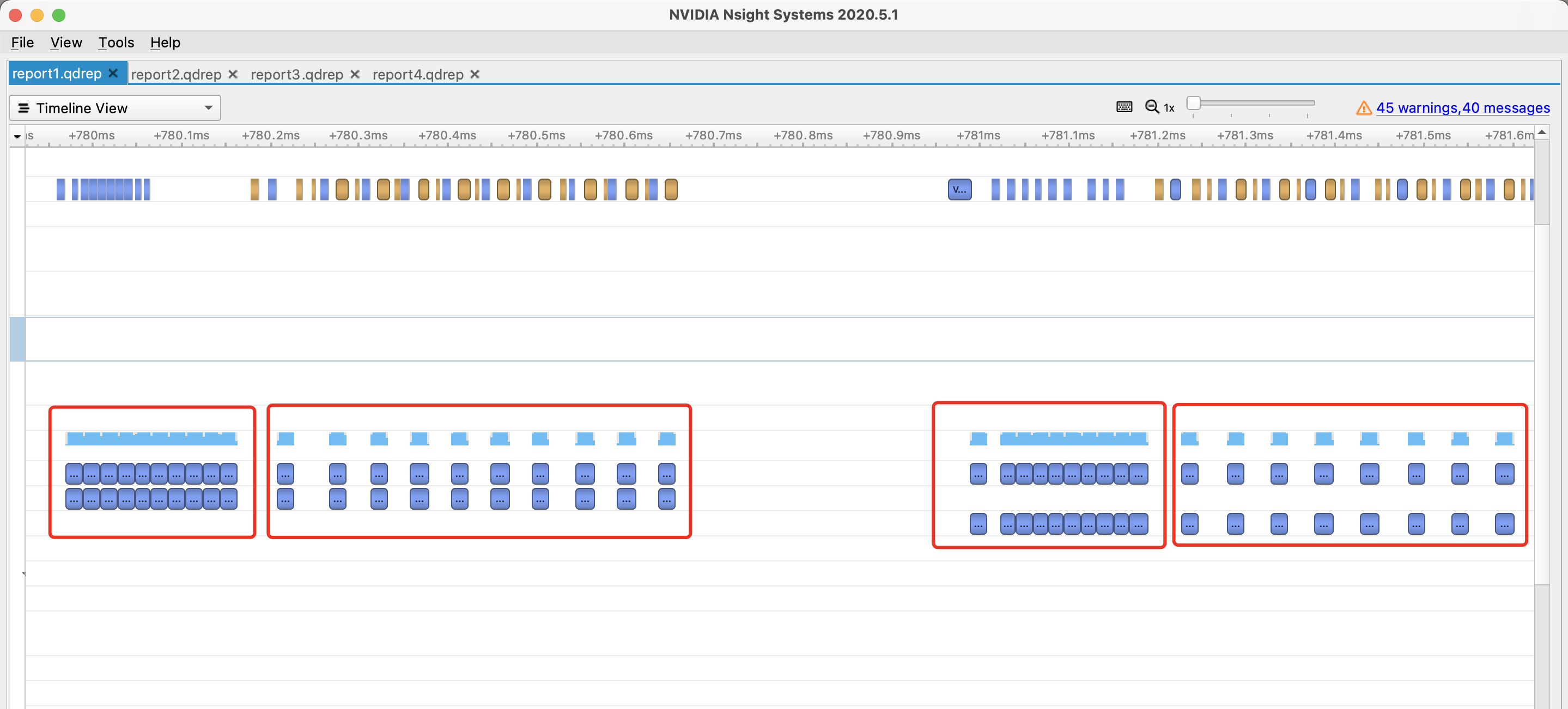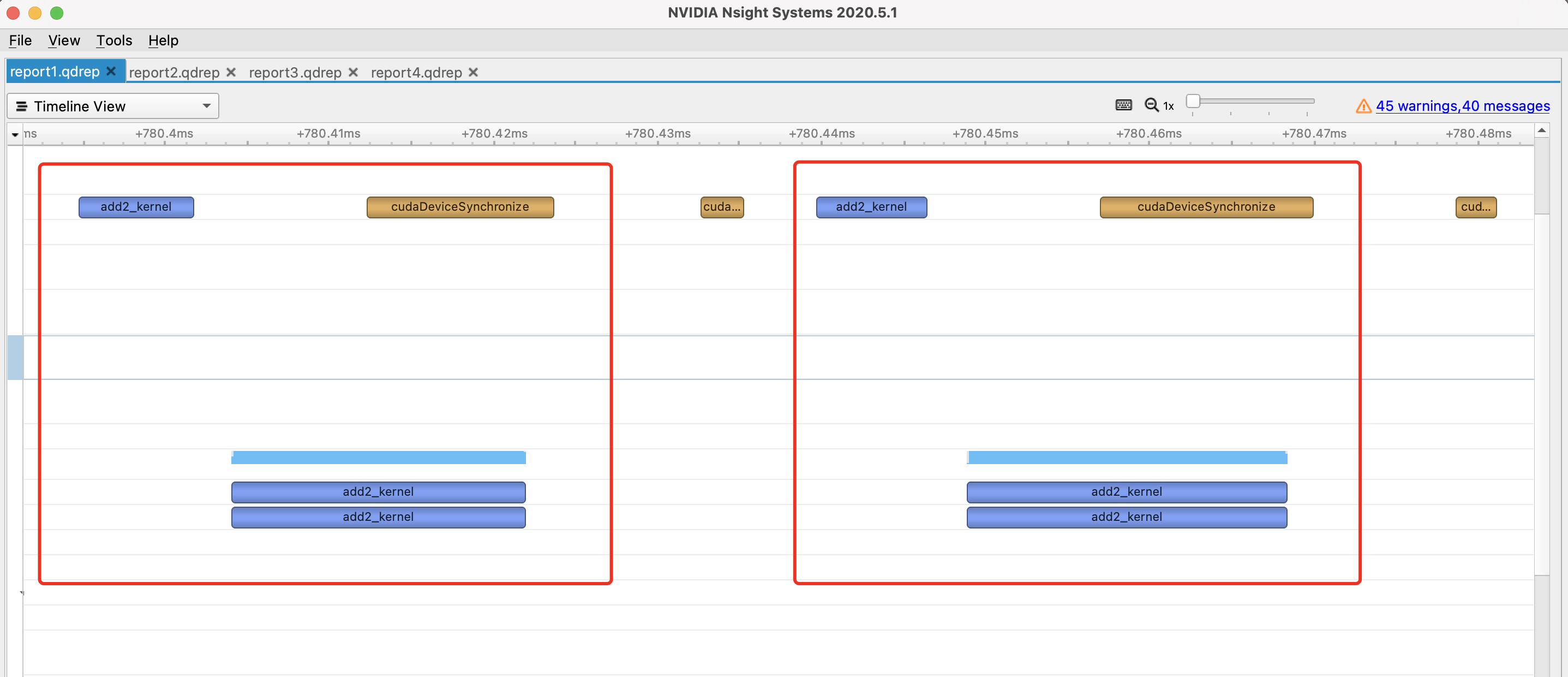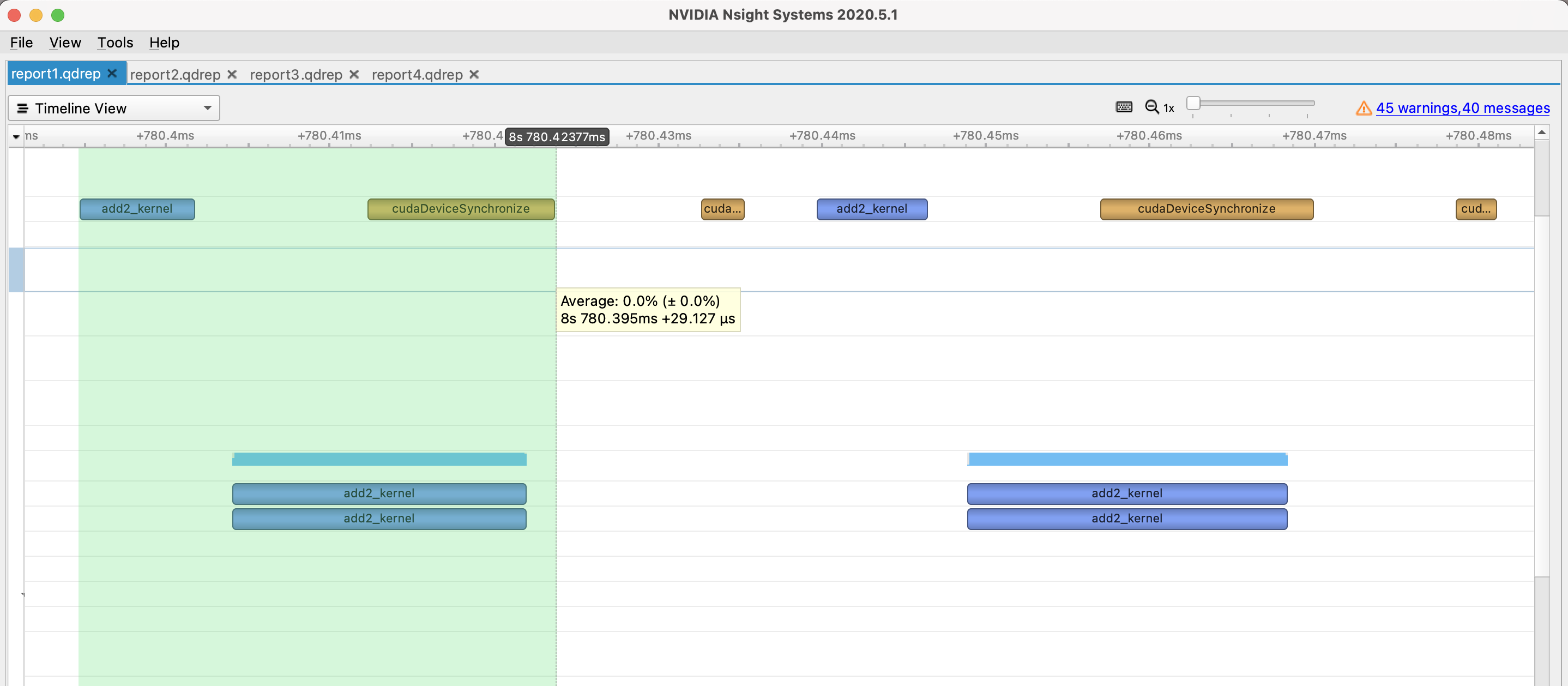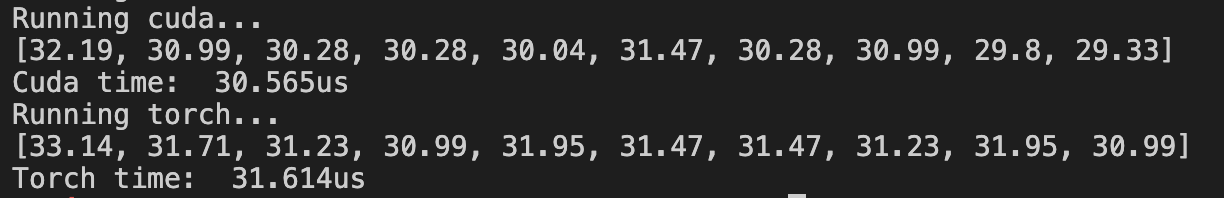### 第一次同步，第二次不同步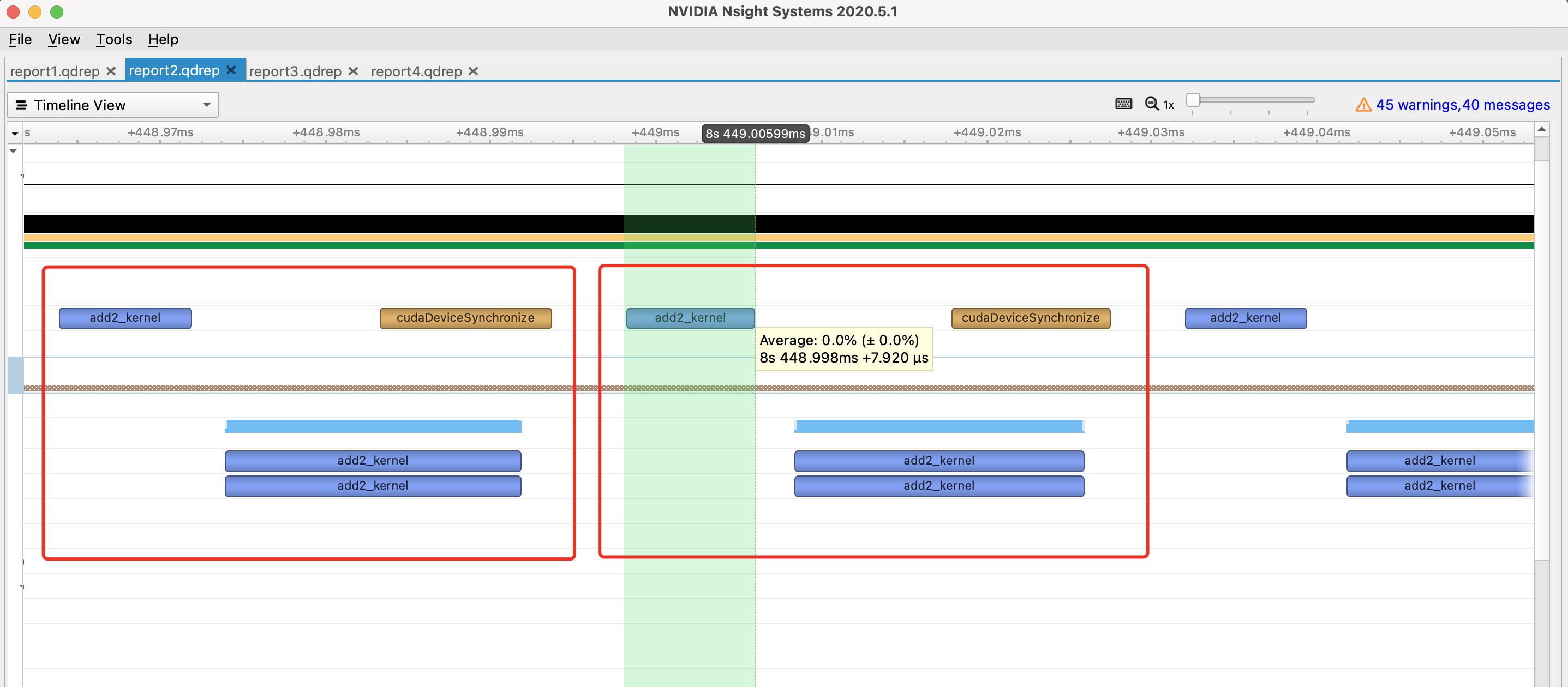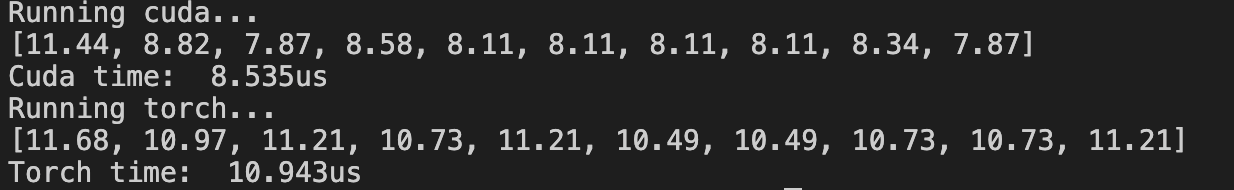### 第一次不同步，第二次同步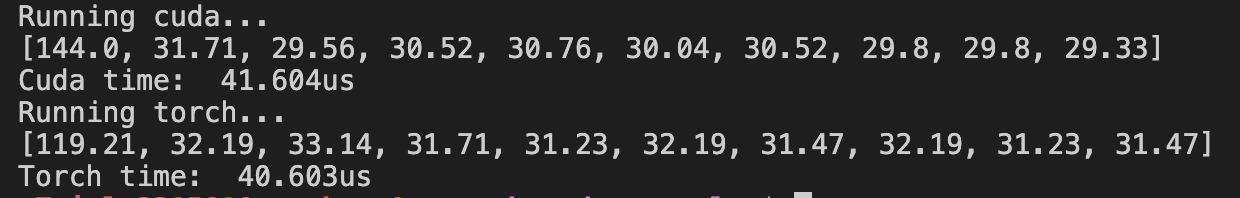### 两次不同步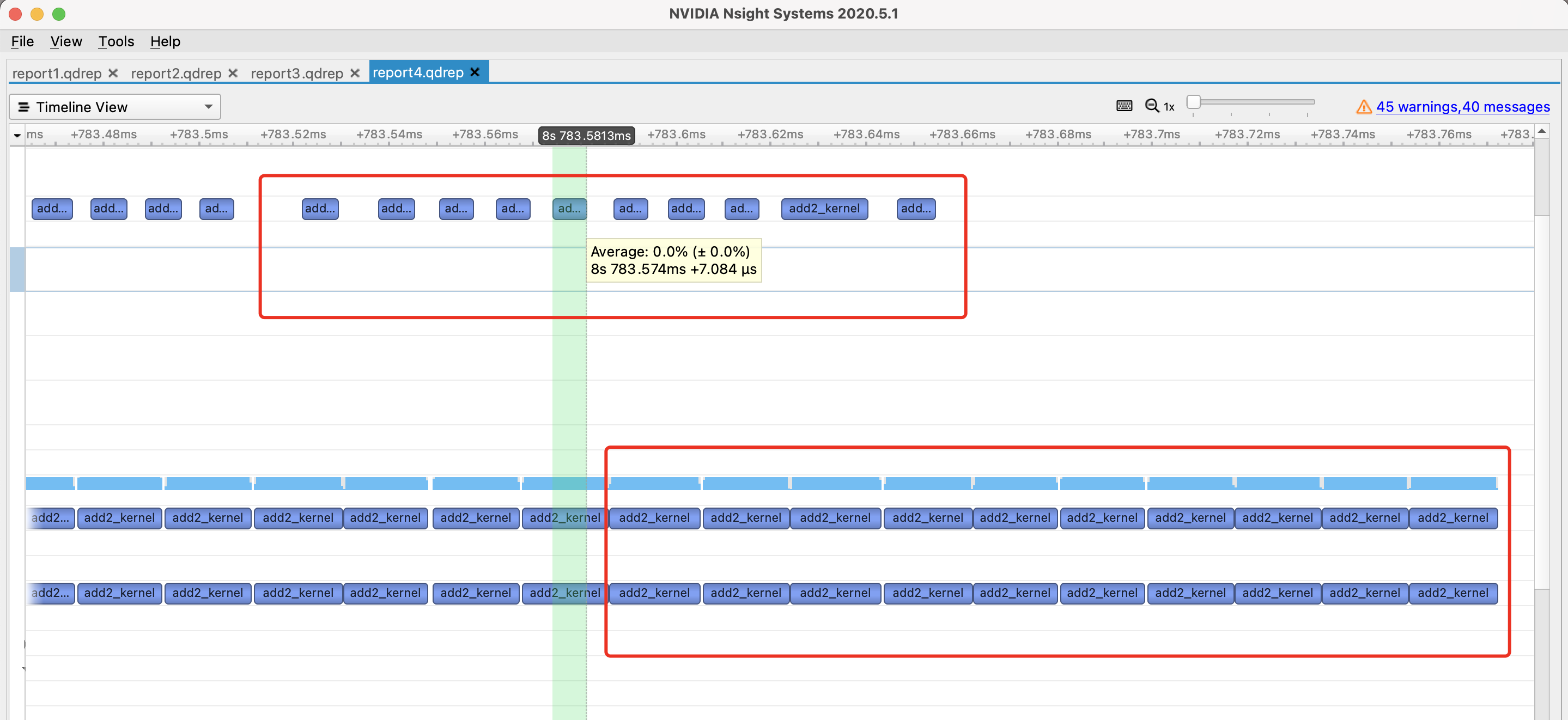## 小结

转载规则

《PyTorch自定义CUDA算子教程与运行时间分析》 韦阳 采用 知识共享署名 4.0 国际许可协议 进行许可。
上一篇详解PyTorch编译并调用自定义CUDA算子的三种方式

2021-03-21微信电脑版重大更新，可以上班刷朋友圈摸鱼了

2021-03-13
目录